• Anna（陈黎娜）

•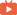词汇（一） 免费
•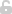词汇（二） 免费
•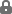词汇（三）
•词汇（四）
•词汇（五）
•词汇（六）
•翻译（一）
•翻译（二）
•翻译（三）
•翻译（四）
•全真模拟试题讲解（一）
•全真模拟试题讲解（二）
•全真模拟试题讲解（三）
•全真模拟试题讲解（四）
•全真模拟试题讲解（六）
•全真模拟试题讲解（七）
•全真模拟试题讲解（五）
•全真模拟试题讲解（八）
•全真模拟试题讲解（九）
•全真模拟试题讲解（十）
•全真模拟试题讲解（十一）
•全真模拟试题讲解（十二）
•全真模拟试题讲解（十三）
•全真模拟试题讲解（十四）
•完成对话（一）
•完成对话（二）
•完成对话（三）
•写作（一）
•写作（二）
•语法（一）
•语法（二）
•语法（三）
•语法（四）
•语法（五）
•语法（六）
•语法（七）
•语法（八）
•语法（九）
•语法（十）
•语法（十一）
•语法（十二）
•语法（十三）
•语法（十四）
•阅读理解（一）
•阅读理解（二）
•阅读理解（三）
•阅读理解（四）
•阅读理解（五）
•阅读理解（六）
•阅读理解（七）
•阅读理解（八）
•阅读理解（九）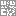免费领取更多课程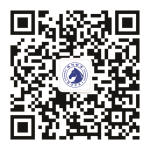0条评论

课程介绍

### 2022年学位英语（三级）-精讲班

有  效  期：2023-06-26
主  　  讲：Anna（陈黎娜）    年份：1 年
课程推荐 更多课程